# 11. Normed linear spaces.

A collection V  of objects (functions, vectors,…) is called a real linear space if

a) there is an operation + on V  such that if u and v  belong to V  then so does also u+v,

b) the operation +  is commutative: u+v=v+u,

c) the operation +  is associative: u+(v+w)=(u+v)+w,

d) there is a zero element 0 in V  such that u+0=u  for all u in V.

e) for every u  in V  there is an inverse denoted -u  such that u+(-u)=0,

f) for all u  in V  and all a  in R the scalar multiplication au  is defined and belongs to V,

g) the scalar multiplication is associative and distributive:

a(bu)=(ab)u,
(a+b)u=au+bu,
a(u+v)=au+av,
for all a,b in R and all u,v in V,

h) 1u=u for all u in V.

Example 17: The set Rn.
The objects are the n-tipels x=(x1,x2,…,xn)  where xk  belong to R. The operation + is defined viaScalar multiplication ax  is defined via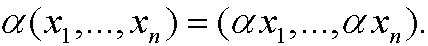We have zero element (0,0,…,0)  and an inverse -x=(-x1,-x2,…,-xn).

Example 18: The set C[a,b].
The objects are all continuous functions f  on [a,b]. The operation +  is defined via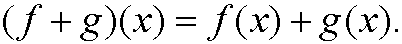Scalar multiplication af  is  defined viaWe have a zero element f(x)=0  and an inverse -f(x) for all x  in [a,b].

Example 19: The set Cn[a,b]. Analogously as example 18 above.

Example 20: The set V  of all 2×2 matrices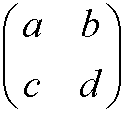such that ad-bc=0  is not a linear space.

Proof: It is enough to find one counter example. Pick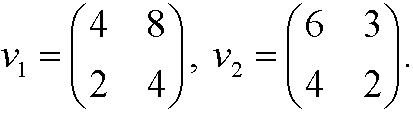Then both v1  and v2  belong to the space V  butWe conclude that a) is not fulfilled.

normed linear space is a linear spaceV  equipped with a norm. A norm is a mapping that to every element y  in V  maps a nonnegative number ||y||that fulfills the conditionsExample 21: Rn equipped with either of the norms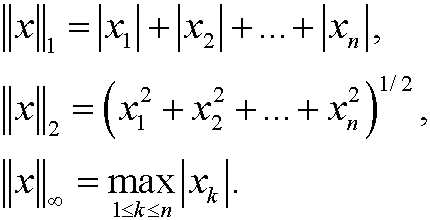Example 22: C[a,b] equipped with either of the norms<>We get the distance d(y1,y2) between two functions y1  and y2  for a given norm by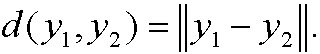This means that a norm always induce a corresponding way to measure distance between objects in a space.